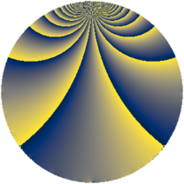# Properties

 Label 115.2.jLevel $115$ Weight $2$ Character orbit 115.j Rep. character $\chi_{115}(4,\cdot)$ Character field $\Q(\zeta_{22})$ Dimension $100$ Newform subspaces $1$ Sturm bound $24$ Trace bound $0$

# Related objects

## Defining parameters

 Level: $$N$$ $$=$$ $$115 = 5 \cdot 23$$ Weight: $$k$$ $$=$$ $$2$$ Character orbit: $$[\chi]$$ $$=$$ 115.j (of order $$22$$ and degree $$10$$) Character conductor: $$\operatorname{cond}(\chi)$$ $$=$$ $$115$$ Character field: $$\Q(\zeta_{22})$$ Newform subspaces: $$1$$ Sturm bound: $$24$$ Trace bound: $$0$$

## Dimensions

The following table gives the dimensions of various subspaces of $$M_{2}(115, [\chi])$$.

Total New Old
Modular forms 140 140 0
Cusp forms 100 100 0
Eisenstein series 40 40 0

## Trace form

 $$100q - 14q^{4} - 9q^{5} - 18q^{6} - 12q^{9} + O(q^{10})$$ $$100q - 14q^{4} - 9q^{5} - 18q^{6} - 12q^{9} - 13q^{10} - 26q^{11} - 26q^{14} - 10q^{15} - 18q^{16} - 14q^{19} + 49q^{20} - 22q^{21} - 68q^{24} + 21q^{25} - 42q^{26} - 24q^{29} + 19q^{30} - 12q^{31} + 8q^{34} - 37q^{35} - 10q^{36} + 14q^{39} - q^{40} + 8q^{41} + 166q^{44} - 42q^{45} - 18q^{46} + 32q^{49} - 23q^{50} - 22q^{51} + 116q^{54} + 27q^{55} - 116q^{56} + 50q^{59} + 123q^{60} - 38q^{61} + 10q^{64} + 76q^{65} - 28q^{66} + 80q^{69} + 102q^{70} - 110q^{71} + 22q^{74} + 6q^{75} + 4q^{76} + 42q^{79} + 18q^{80} + 204q^{81} + 56q^{84} - 121q^{85} + 132q^{86} - 66q^{89} - 198q^{90} + 76q^{91} - 70q^{94} - 74q^{95} + 236q^{96} - 60q^{99} + O(q^{100})$$

## Decomposition of $$S_{2}^{\mathrm{new}}(115, [\chi])$$ into newform subspaces

Label Dim. $$A$$ Field CM Traces $q$-expansion
$$a_2$$ $$a_3$$ $$a_5$$ $$a_7$$
115.2.j.a $$100$$ $$0.918$$ None $$0$$ $$0$$ $$-9$$ $$0$$